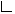SWIXML
1.6 (#161)

## org.swixml Class ConverterLibrary

```java.lang.Objectorg.swixml.ConverterLibrary
```

`public class ConverterLibraryextends Object`

The `ConverterLibrary` contains all available Coverters, converting Strings.

General purpose data type converters are able to convert Strings into objects that are usually as parameters when setters are called on javax.swing objects.

``` Available Converter include converters able to produce

Primitives
Dimension
Color
Border
etc.

```
``` Example String inputs could look like this:

MatteBorder(4,4,4,4,red)
FFCCEE
BorderLayout.CENTER
2,2,2,2

```
Date: Dec 16, 2002

Version:
\$Revision: 1.1 \$
Author:
Wolf Paulus
`Converter`

Method Summary
` Converter` `getConverter(Class template)`
Returns a `Converter` instance, able to produce objects of the given `class`
` Map` `getConverters()`

`static ConverterLibrary` `getInstance()`

` boolean` `hasConverter(Class template)`
Indicates if a the ConverterLibary has a Converter producing instances of the given Class.
` void` ```register(Class template, Converter converter)```
Registers a Converter with the ConverterLibrary
` void` `register(Converter converter)`
Registers a Converter with the ConverterLibrary

Methods inherited from class java.lang.Object
`clone, equals, finalize, getClass, hashCode, notify, notifyAll, toString, wait, wait, wait`

Method Detail

### getInstance

`public static ConverterLibrary getInstance()`
Returns:
`ConverterLibrary` the single instacne of the ConverterLibrary.

### getConverters

`public Map getConverters()`
Returns:
`Map` - all registered converters.
`Use a class to get to the converters`

### register

`public void register(Converter converter)`
Registers a Converter with the ConverterLibrary

Parameters:
`converter` - `Converter` Instance of Converter able to convert Strings into objects of the given type

### register

```public void register(Class template,
Converter converter)```
Registers a Converter with the ConverterLibrary

Parameters:
`template` - `Class` type of the objects the Converter creates
`converter` - `Converter` Instance of Converter able to convert Strings into objects of the given type

### hasConverter

`public boolean hasConverter(Class template)`
Indicates if a the ConverterLibary has a Converter producing instances of the given Class.

Parameters:
`template` - `Class`
Returns:
`boolean` true, if the ConverterLibrary has a Converter to produce an instances of the gioven class.

### getConverter

`public Converter getConverter(Class template)`
Returns a `Converter` instance, able to produce objects of the given `class`

Parameters:
`template` - `Class` Class of the object the `Converter` needs to produce.
Returns:
`Converter` - instance of the given Converter class.

SWIXML
1.6 (#161)